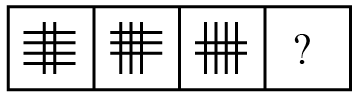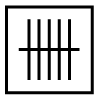Question 10

# Select the figure that can replace the question mark (?) in the following series.Solution

In the given series, the horizontal lines are decreasing by 1 and the vertical lines are increasing by 1. In the next figure, the number of horizontal lines will be 1 and the number of vertical lines will be 5.

$$\therefore\$$The next figure in the series isHence, the correct answer is Option D

• Free SSC Study Material - 18000 Questions
• 230+ SSC previous papers with solutions PDF